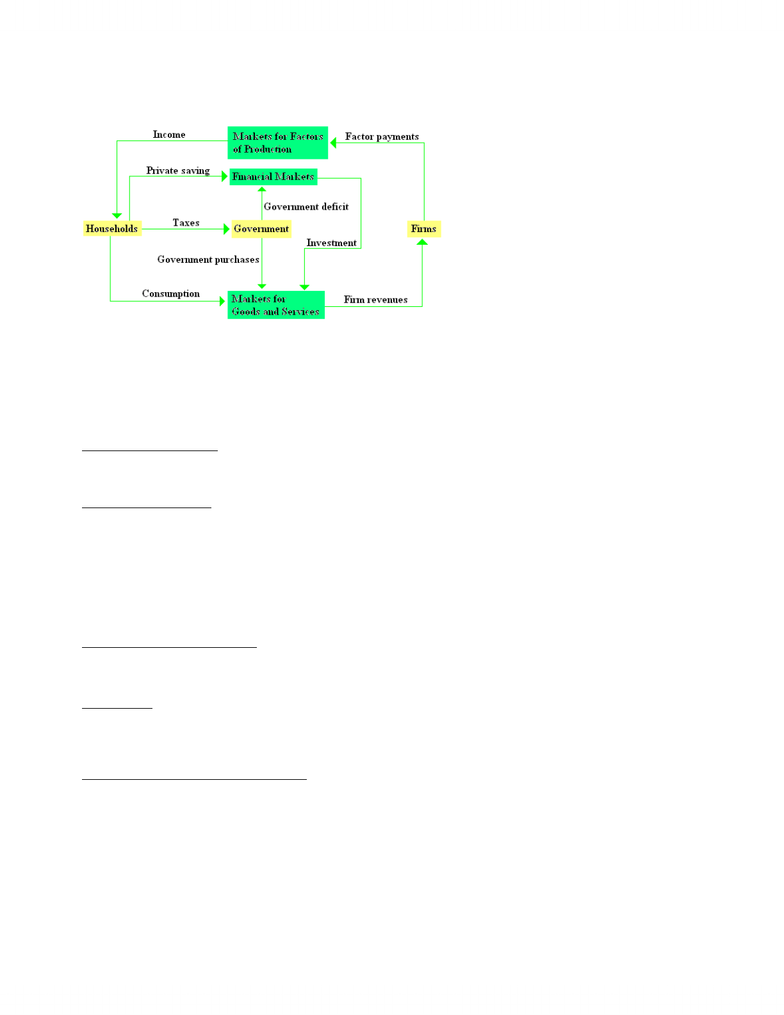Textbook Notes (280,000)
CA (170,000)
UTSC (20,000)
Chapter

# Week 2 chapter notes

Department
Economics for Management Studies
Course Code
MGEB06H3
Professor
Jack Parkinson

This preview shows page 1. to view the full 4 pages of the document.Chapter 3 National Income: Where It Comes From and Where It Goes Notes
The Circular Flow of Dollars Through the Economy
x households receive income and use it to pay taxes to government, to consume goods and services, to save via financial markets
x firms receive revenue from sale of goods and services and use it to pay for the factors of production
x both households and firms borrow in financial markets to buy investment goods, such as housing, and factories
x the government receives revenue from taxes, uses it to pay for government purchases (G)
x excess of tax revenues over G is called public saving, which can be either positive (budget surplus) or negative (budget deficit)
3.1 What Determines the Total Production of Goods and Services?
x an economy’s output of goods and services—its GDP—depends on (1) its quantity of inputs, called the factors of product, and
(2) its ability to turn inputs into output, as represented by the production function
The Factors of Production
x factor of production (FOP) Æ an input used to produce goods and services; for example, capital (K) or labour (L)
x capital is the set of tools that worker’s use, while labour is the time people spend working
x capital can be divided into 2 components—physical capital (such as machines) and knowledge (referred to as human capital)
The Production Function
x the available production technology determines how much output is produced from given amounts of capital and labour
x production function Æ the mathematical relationship showing how the quantities of the factors and production determine the
quantity of goods and services produced; for example, Y = F (K, L)
x the production function reflects the available technology for turning capital and labour into output
x if someone invents a better way to produce a good, the result is more output from the same amounts of capital and labour
x constant returns to scale Æ a property of a production function whereby a proportionate increase in all factors of production
leads to an increase in output of the same proportion
x mathematically, a production function has constant returns to scale if: zY = F (zK, zL) for any positive number z
x this says that if both amount of capital and the amount of labour are multiplied by some number z, output is also multiplied by z
The Supply of Goods and Services
x FOP and production function together determine quantity of goods and services supplied, which in turn equals economy’s output
x Y = F (fixed K, fixed L) = fixed Y
3.2 How is National Income Distributed to the Factors of Production?
Factor Prices
x factor price Æ the amount paid for one unit of a factor of production
x the rental price each FOP receives for its services is in turn determined by the supply and demand for that factor
x regardless of the factor price, the quantity of the factor supplied to the market is the same
x intersection of the downward-sloping factor demand curve and the vertical supply curve determines the equilibrium factor price
The Decisions Facing the Competitive Firm
x a competitive firm is small relative to the markets in which it trades, so it has little influence on market prices
x the competitive firm takes the price of its output and its input as given by market conditions
x to make its product, the firm needs two factors of production, capital, and labour
x the goal of the firm is to maximize profit
x revenue equals P × Y, the selling price of the good P multiplied by the amount of the good the firm produces Y
x costs include both labour costs (W × L, the wages W times the amount of labour L) and capital costs (R × K, the rental price of
capital R times the amount of capital K)
x Profit = Revenue – Labour Costs – Capital Costs = PY – WL – RK
x to see how profit depends on the factors of production, substitute the production function Y = F (K, L) for Y to obtain:
Profit = PF(K, L) – WL – RK
x this equation shows that profit depends on the product price P, the factor prices W and R, and the factor quantities L and K
www.notesolution.com